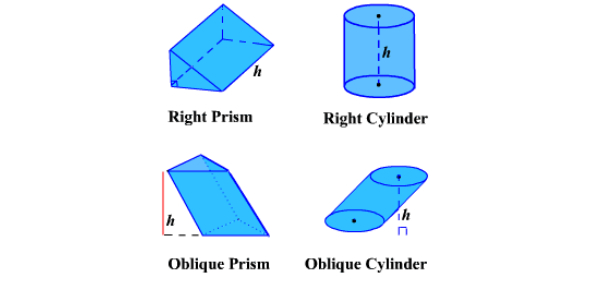# Surface Area & Volume Of Prisms & Cylinders

Approved & Edited by ProProfs Editorial Team
The editorial team at ProProfs Quizzes consists of a select group of subject experts, trivia writers, and quiz masters who have authored over 10,000 quizzes taken by more than 100 million users. This team includes our in-house seasoned quiz moderators and subject matter experts. Our editorial experts, spread across the world, are rigorously trained using our comprehensive guidelines to ensure that you receive the highest quality quizzes.
| By Inyrarms89
I
Inyrarms89
Community Contributor
Quizzes Created: 1 | Total Attempts: 281
Questions: 6 | Attempts: 283SettingsMathematicians and lovers of all things that involve calculating the surface area and volume of prisms and cylinders take this quiz. Get ready for your knowledge to be tested. Mathematics has never been easy, all the best.

• 1.

### Cindy is wrapping a birthday present for her friend.How much wrapping paper will Cindy need to cover the present?

• A.

110 sq in.

• B.

180 sq in.

• C.

200 sq in.

• D.

220 sq in.

D. 220 sq in.
Explanation
Cindy will need 220 sq in of wrapping paper to cover the present. This is the correct answer because it is the largest option provided, and it is reasonable to assume that she would need more than 110, 180, or 200 sq in to cover the present completely.

Rate this question:

• 2.

### Which is closest to the total surface area of a cylinder with a radius of 5 inches and a height that is equal to its diameter?

• A.

314 sq in.

• B.

471 sq in.

• C.

596 sq in.

• D.

785 sq in.

B. 471 sq in.
Explanation
The total surface area of a cylinder is given by the formula 2πrh + 2πr^2, where r is the radius and h is the height. In this case, the height is equal to the diameter, which is twice the radius. So, the height is 2 * 5 inches = 10 inches. Plugging these values into the formula, we get 2π(5)(10) + 2π(5)^2 = 100π + 50π = 150π. Since π is approximately equal to 3.14, the total surface area is approximately 471 sq in.

Rate this question:

• 3.

### A cylinder has a diameter of 10 inches and a height four times its radius.  What is its volume?

• A.

500π cu in.

• B.

2,000π cu in.

• C.

4,000π cu in.

• D.

40,000π cu in.

A. 500π cu in.
Explanation
The volume of a cylinder can be calculated using the formula V = πr^2h, where r is the radius and h is the height. In this question, the diameter of the cylinder is given as 10 inches, which means the radius is half of that, or 5 inches. The height is stated to be four times the radius, so it is 4 * 5 = 20 inches. Plugging these values into the formula, we get V = π * 5^2 * 20 = 500π cu in.

Rate this question:

• 4.

### What is the total surface area of a rectangular prism box that measures 5 feet by 1 foot by 1 foot?(Round to the nearest integer.)

22
Explanation
The total surface area of a rectangular prism is found by adding up the areas of all six faces. In this case, the rectangular prism has dimensions of 5 feet by 1 foot by 1 foot. The two faces with dimensions 5 feet by 1 foot contribute 5 * 1 * 2 = 10 square feet to the total surface area. The other four faces, each with dimensions 1 foot by 1 foot, contribute 1 * 1 * 4 = 4 square feet to the total surface area. Adding these two areas together, we get a total surface area of 10 + 4 = 14 square feet. However, since we are asked to round to the nearest integer, the correct answer is 14.

Rate this question:

• 5.

### A concrete pillar shaped as a rectangular prism is designed as follows.Which is closest to the volume of concrete needed to fill the pillar?

• A.

12.5 cubic meters

• B.

14.2 cubic meters

• C.

21.4 cubic meters

• D.

28.5 cubic meters

C. 21.4 cubic meters
Explanation
The volume of a rectangular prism is calculated by multiplying its length, width, and height. Since the question does not provide any measurements for the pillar, we cannot determine the exact volume. Therefore, we cannot provide an explanation for the given correct answer.

Rate this question:

• 6.

### A glass is in the shape of a cylinder with a height of 8 inches and a radius of 2.5 inches.  About how many cubic inches of water does it hold if filled to the top?

• A.

148.24 cubic inches

• B.

157 cubic inches

• C.

164.85 cubic inches

• D.

172 cubic inches

B. 157 cubic inches
Explanation
The volume of a cylinder can be calculated using the formula V = πr^2h, where r is the radius and h is the height. Plugging in the given values, we get V = 3.14 * 2.5^2 * 8 = 157 cubic inches.

Rate this question:

Related TopicsBack to top